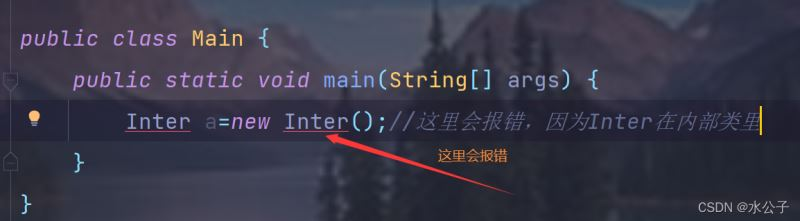﻿ JavaSE学习之内部类及常用API_java_脚本之家
java# JavaSE学习之内部类及常用API

## 1.内部类

### 1.1 内部类概述

```public class Outer{
public class Inter{//内部类
}
}
```

1. 内部类可以直接访问外部类成员，包括私有

2. 外部类如果想访问内部类就要创建对象

```public class Outer {//创建外部类
private  int age=9;
public class Inter{//创建内部类
//内部类创建方法
public void show(){
//内部类可直接访问外部类
System.out.println(age);
}
}
public void method(){
//这里是外部类的调用，那么不能直接像下面一样调用show()方法，
//不然就会报错，不能直接访问
//show();不能这样
//应改为创建内部类对象，通过对象进行访问
Inter i=new Inter();
i.show();
}
}
```

### 1.2 成员内部类

• 在类的成员位置：成员内部类
• 在类的局部位置：局部内部类
```//类
public class Outer {//创建外部类
private  int age=9;
public class Inter{//创建内部类
//内部类创建方法
public void show(){
//内部类可直接访问外部类
System.out.println(age);
}
}
}
```
```//测试类

```• `外部类.内部类 对象名=new 外部类.new内部类();`
• `Outer.Inter oi=new Outer().new Inter();`
```//测试类
public class Main {
public static void main(String[] args) {
Outer.Inter oi=new Outer().new Inter();//构造内部类对象
oi.show();
}
}
```

```//类
public class Outer {//创建外部类
private  int age=9;
private class Inter{//创建内部私有类
//内部类创建方法
public void show(){
//内部类可直接访问外部类
System.out.println(age);
}
}
public void method(){
Inter i=new Inter();//创建内部类对象间接访问
i.show();  //调用方法
}
}
```

9

### 1.3 局部内部类

```//类
public class Outer {//创建外部类
private int age = 9;

public void method() { //在方法中局部创建内部类

class Inter {//创建内部类
private int age2 = 2;//局部变量也可以

public void show() {
System.out.println(age);
System.out.println(age2);
}
}
Inter i = new Inter();//创建内部类对象间接访问
i.show();  //调用方法
}
}
```
```public class Main {
public static void main(String[] args) {
Outer o=new Outer();
o.method();//间接调用方法
}
}

```

### 1.4 匿名内部类

• 前提：存在一个类或者接口，这里的类可以是具体类，也可以是抽象类
• 格式：`new 类名或者接口名(){重写方法}`
• 范例：`new Inter(){public void show(){}};`
• 解释： new Inter()是匿对象，继承了这个类，或实现了这个接口
• 本质： 是一个继承了该类或者实现了该接口的子类匿名对象,

```//前提：一个类或者接口
public interface Inter {//写接口
void show();//写抽象方法
}
```

```public class Outer {//创建外部类

public void method() {
new Inter() {//这个是对象
@Override//重写方法
public void show() {
System.out.println("匿名内部类");
}
}.show();//通过对象调用方法
}
}
```

```//测试类
public class Main {
public static void main(String[] args) {
Outer o=new Outer();
o.method();//调用方法
}
}

```

```public class Outer {//创建外部类

public void method() {
Inter i =new Inter() {//多态形式，左面接口名，右面对象
@Override//重写方法
public void show() {
System.out.println("匿名内部类");
}
};
i.show();//遵循编译看左面，执行看右面
i.show();
}
}
```

### 1.5 匿名内部类在开发中的使用

```//接口
public interface Jummping {
void jump();//抽象类
}
```
```//操作类
public class JumppingOperator {
public void useOperator(Jummping j){//接口名作为形参，new Jumpping(){}
j.jump();//内部类对象调用方法
}

```
```public class Jumpping {
public static void main(String[] args) {
JumppingOperator j = new JumppingOperator();//创建操作类对象
j.useOperator(new Jummping() {
@Override
public void jump() {
System.out.println("猫跳高了");
}
});//用匿名内部类实现
//重复使用，就不用再创建Cat类或者Dog类了
j.useOperator(new Jummping() {
@Override
public void jump() {
System.out.println("狗跳墙了");
}
});
}
}
```

## 2.常用API

### 2.1Math

public final class Math extends Object被final修饰，是最终类，详细内容见APi手册```public class Main {
public static void main(String[] args) {
//绝对值
System.out.println(Math.abs(-88));
System.out.println(Math.abs(88));
System.out.println("----------");
//返回大于或者等于参数的double值为一个整数,（向上取整）
System.out.println(Math.ceil(1.12));
System.out.println("----------");
//返回0小于或者等于参数的double值为一个整数,（向下取整）
System.out.println(Math.floor(1.21));
System.out.println("----------");
//返回最大值
System.out.println(Math.max(1,2));
System.out.println("----------");
//返回最小值
System.out.println(Math.min(2,6));
System.out.println("----------");
//返回a的b次幂
System.out.println(Math.pow(2,2));
System.out.println("----------");
//返回四舍五入的整数值
System.out.println(Math.round(1.51));
System.out.println("---------");
//返回随机值，[0-1.0)
System.out.println(Math.random());
//返回1-100整数随机值
System.out.println((int)(Math.random()*100)+1);

}
} ```

88

88

----------

2.0

----------

1.0

----------

2

----------

2

----------

4.0

----------

2

---------

0.6041376042702374

78

### 2.2 System```public class Main {
public static void main(String[] args) {
System.out.println("start");
System.exit(0);
//java虚拟机已经停止运行，无法在进行执下面的语句
System.out.println("end");
}
}
```

start

```public class Main {
public static void main(String[] args) {
System.out.println(System.currentTimeMillis()*1.0/1000/60/60/24/365+"年");
System.out.println("开始计算下面程序执行的时间");
long start=System.currentTimeMillis();//用lang定义，因为数字太大
for(int i = 0; i <10000;i++){
System.out.println(i);
}
long end=System.currentTimeMillis();//用lang定义
System.out.println("程序运行时间为："+(end-start)+"毫秒");
}
}

```

### 2.3 Object

Object是类层次结构的根，每个类都可以将Object作为超类。所有类都直接或者间接的继承自该类

toString()方法的使用

```public class Student {//其实这里继承了Object,所以Student这个类理论上是子类，
//所以可以使用toString()方法，但要重写
private String name;
private  int age;

public Student() {
}

public Student(String name, int age) {
this.name = name;
this.age = age;
}

public String getName() {
return name;
}

public void setName(String name) {
this.name = name;
}

public int getAge() {
return age;
}

public void setAge(int age) {
this.age = age;
}

@Override
public String toString() {//重写方法
return "Student{" +
"name='" + name + '\'' +
", age=" + age +
'}';
}
}
``````//测试类
public class Main {
public static void main(String[] args) {
Student s=new Student("张三",18);//采用有参构造
System.out.println(s);//因为使用了toString()方法，
// 就不用再用getName(),getAge()输出了，直接输出s

}
} ```

Student{name='张三', age=18}

equal()方法```//测试类
public class Main {
public static void main(String[] args) {
Student s1=new Student("张三",18);//采用有参构造
System.out.println(s1);
Student s2=new Student("张三",18);//采用有参构造
System.out.println(s2);
System.out.println(s1.equals(s2));
//这里因为是new出来的，所以比较地址就会返回false，那么只有重写了方法才会

}
}
```
```//学生类
public class Student {
private String name;
private  int age;

public Student() {
}

public Student(String name, int age) {
this.name = name;
this.age = age;
}

public String getName() {
return name;
}

public void setName(String name) {
this.name = name;
}

public int getAge() {
return age;
}

public void setAge(int age) {
this.age = age;
}
@Override
public boolean equals(Object o) {
if (this == o) return true;
if (o == null || getClass() != o.getClass()) return false;

Student student = (Student) o;

if (age != student.age) return false;
return name != null ? name.equals(student.name) : student.name == null;
}

}

```### 2.4 Array的排序1. 构造方法用private修饰：为了防止外界创建对象

2. 成员用public static修饰：使用类名来访问该成员变量

```import java.util.Arrays;

public class Main {
public static void main(String[] args) {
int []arr={2,7,3,4,9,5};
System.out.println("排序前: "+Arrays.toString(arr));
Arrays.sort(arr);
System.out.println("排序后: "+Arrays.toString(arr));

}
} ```

```public class Main {
public static void main(String[] args) {

Scanner sc=new Scanner(System.in);
System.out.println("请你输入一个数n:");
int []arr=new int[sc.nextInt()];//动态数组
for(int i=0;i< arr.length; i++){
arr[i]=sc.nextInt();
}
Arrays.sort(arr);
System.out.println("排序后:"+Arrays.toString(arr));
}
} ```

5

1 5 3 7 9

[1, 3, 5, 7, 9]